Home > GC > Chapter 8 > Lesson 8.2.1 > Problem8-68

8-68.
1.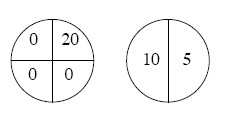Your teacher has offered your class extra credit. She has created two spinners, shown at right. Your class gets to spin only one of the spinners. The number that the spinner lands on is the number of extra credit points each member of the class will get. Study both spinners carefully.Homework Help ✎

1. Assuming that each spinner is divided into equal portions, which spinner do you think the class should choose to spin and why?

2. What if the spot labeled “20” were changed to “100”? Would that make any difference?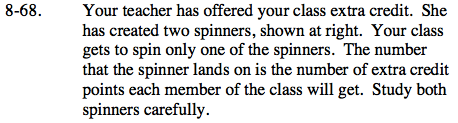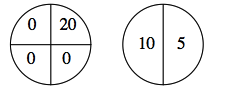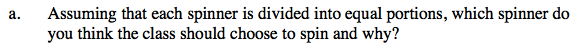$\text{The spinner on the left earns 20 points only }\frac{1}{4}\text{ of the time.}$

$\text{The spinner on the right earns 10 points }\frac{1}{2}\text{ of the time and 5 points }\frac{1}{2}\text{ of the time.}$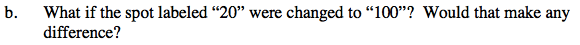$\text{Now the spinner on the left earns 100 points }\frac{1}{4}\text{ of the time.}$